# How to write subscript(x₂) in LaTeX?

Subscripts are commonly used when creating a document. In this tutorial we will discuss how to use subscripts in LaTeX, both in text mode and math mode.

## Use subscript in math mode

Using subscripts in math mode is very easy, you just need to use this character _(underscore). For example if you want to print O₂ then you have to write the syntax like this $O_2$.

\documentclass{article}
\usepackage{amsmath} % For \longrightarrow
\begin{document}
$\verb|H_2 O|\longrightarrow H_2 O$
$\Lambda_0\quad\Lambda_e\quad\Lambda_\nu$
$\sum_n \quad \bigcup_m\quad \int_0$
$(a_0,a_1,a_2,\dots,a_n)$
\end{document}

Output :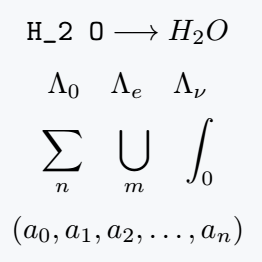If you want to use more than one character in the subscript, pass the characters in curly brackets {}.

Moreover, the first characters will be printed as subscript and the remaining characters will be printed as normal text.

So if you want to print An+1 then the syntax will be A_{n+1}.

\documentclass{article}
\usepackage{amsmath} % For \longrightarrow
\begin{document}
$\verb|A_n+1|\longrightarrow A_n+1$
$\verb|A_{n+1}|\longrightarrow A_{n+1}$
$\Lambda_{pq}\quad\Lambda_{\mu e}\quad\Lambda_{\nu a}$
$\sum_{n=1} \quad \bigcup_{m=1}\quad \int_{2\pi}$
$(a_0,a_1,a_2,\dots,a_{(n-1)},a_n)$
\end{document}

Output :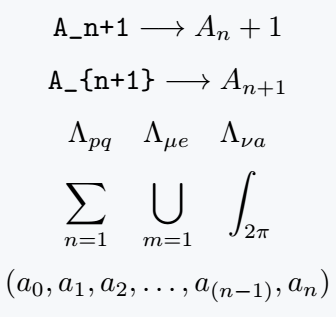Also you can use single dollar to use superscript in inline mode. Like this $O_2$

\documentclass{article}
\begin{document}
What is $O_2$ used for?\\[4pt]
What is $H_2 SO_4$ called in chemistry?\\[4pt]
Summation in Mathematics is denoted by the symbol $\sum$ and for a set of real numbers $x_1, x_2, \cdots, x_n$, their sum is defined as $\sum_{k=1}^{n}x_k$ .  That is
\end{document}

Output :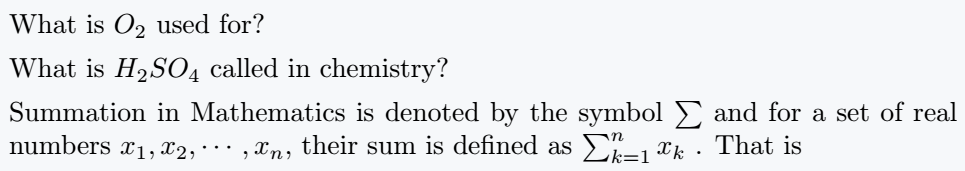## Subscript in usual text mode

LaTeX provides a default command to use subscripts in the text mode of LaTeX, which is \textsubscript. With this command you can easily use subscript in usual text mode.

\documentclass{article}
\usepackage{amsmath} % For \longrightarrow
\begin{document}
\verb|A\textsubscript{n-1}|$\longrightarrow$ A\textsubscript{n-1}\\[4pt]
What is O\textsubscript{2} used for?\\[4pt]
What is H\textsubscript{2}SO\textsubscript{4} called in chemistry?\\[4pt]
(a\textsubscript{0},a\textsubscript{1},a\textsubscript{2},$\dots$,a\textsubscript{n-1},a\textsubscript{n})
\end{document}

Output :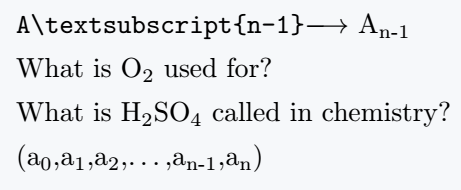## Multiple(double) subscript in LaTeX

If you want to use double subscripts, write the syntax a_{b_c } or {a_b}_c rather than a_b_c .

And if you write like this a_b_c you will get error message. Also you can write triple subscript like this a_{b_{c_d}}.

\documentclass{article}
\usepackage{amsmath} % For \longrightarrow
\begin{document}
\verb|a_{b_c}|$\longrightarrow a_{b_c}$\\[5pt]
\verb|{a_b}_c|$\longrightarrow {a_b}_c$\\[5pt]
\verb|a_{b_{c_d}}|$\longrightarrow a_{b_{c_d}}$\\[5pt]
\verb|a_{1_{2_{3_{\ddots_{n}}}}}|$\longrightarrow a_{1_{2_{3_{\ddots_{n}}}}}$
\end{document}

Output :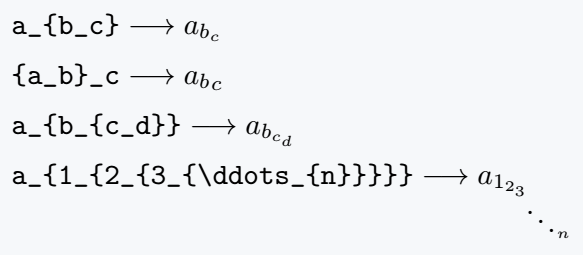#### Md Jidan Mondal

LaTeX expert with over 10 years of experience in document preparation and typesetting. Specializes in creating professional documents, reports, and presentations using LaTeX.